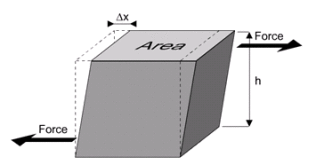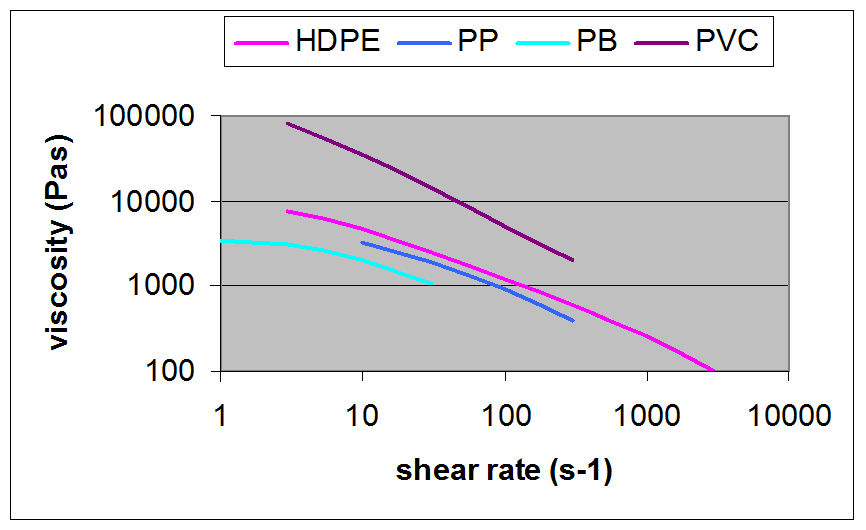# 10. Viscosity (melt phase)

## Definition of viscosity

Consider a cube made from an elastic material, having ribs with length h. The cube is glued to a table. If we would apply a force to the top of the cube parallel to the surface of the table then the top of the cube will be displaced over a distance ∆x, as shown in Figure 43. This kind of deformation is called shear deformation. Its value is the deformation divided by the height ∆x/h. The shear deformation is often denoted by the symbol g. The force acting on the cube divided by the area of the cube (F/h2) is called the shear stressA cube deformed by a shear stress

The ratio between the shear stress and the shear deformation is called the shear modulus. It can be calculated from:
[shear modulus] = [shear stress] / [shear deformation] or [shear stress] = [shear modulus] * [shear deformation]

Now suppose that the cube is made from a fluid like water or molten polymer. If a shear stress would be applied to this cube, then the top of the cube would continuously move in the direction of the stress with a certain velocity as long as the force exists. This continuous deformation is called shear rate and it is defined as the velocity of the top divided by the height.

The ratio between the shear stress and the shear rate is called the viscosity. It can be calculated from:
[viscosity] = [shear stress] / [shear rate] or [shear stress] = [viscosity] * [shear rate]

The viscosity is an important material property of a fluid. With the aid of the viscosity the shear stress can be calculated when the fluid is sheared. Eventually this gives us the possibility to calculate the required pressure to move a fluid through a channel.

## 1.1     How to calculate the viscosity from the differential equation

In chapter 8 we have shown the general outline of the differential equation for the rubber and melt phase:
[change of rubber stress per unit of time] = + [change of rubber stress due to deformation per unit time] – [rubber stress] / [rubber relaxation time]

This differential equation can be used for the calculation of the viscosity from the material properties. The rubber stress is the same as the shear stress. We assume that after some time of shearing the shear stress has become constant. We have a dynamic equilibrium. That means that the change of stress has become zero:
0 = + [change of rubber stress due to deformation per unit time] – [shear stress] / [rubber relaxation time]

The change of rubber stress per unit time is the shear modulus times the shear rate:
[change of rubber stress due to deformation per unit time]= [shear modulus] * [shear rate]

By combining these two equations we get:
0 = [shear modulus] * [shear rate]] – [shear stress] / [relaxation time]

This can be rewritten into:
[shear stress] = [shear modulus] * [relaxation time] * [shear rate]

Remember that the viscosity has been defined as:
[viscosity] = [shear stress] / [shear rate]

Which gives us the following result for the viscosity:
[viscosity] = [shear modulus] * [relaxation time]

By using a differential equation in a dynamic equilibrium, we have proven that the viscosity of a fluid is simply the shear modulus time the relaxation time. Since the relaxation time of a polymer strongly reduces with the applied stress it follows that the viscosity of a polymer will also decrease with increasing shear stress or shear rate. This is a commonly observed phenomenon with polymers as shown in the figure below.## Summary

• Viscosity is a material property of a polymer in the melt phase that relates the stress to the shear rate.
• The viscosity can be derived from the differential equation that describes the relaxation of the stress in the rubber and melt phase. It is only needed to assume a dynamic equilibrium.
• The viscosity is simply the rubber shear modulus times the rubber relaxation time.

Learn more:

Downloaden (PDF, 1.09MB)